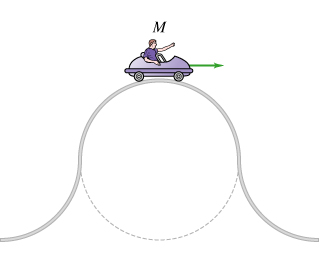# Problem: Six roller-coaster carts pass over the same semicircular"bump." (Intro 1 figure) The mass M of each cart (including passenger) and the normal force n of the track on the cart at the top of each bump are given in the figures.  Rank the speeds of the different carts as each passes over the top of the bump.Rank from largest to smallest. Torank items as equivalent, overlap them.a) 200N, 400kgb) 400N, 100kgc) 300N, 300kgd) 800N, 100kge) 800N, 800kgf) 400N, 200kg

###### FREE Expert Solution

Newton's second law:

$\overline{){\mathbf{\Sigma }}{\mathbf{F}}{\mathbf{=}}{\mathbf{m}}{\mathbf{a}}}$

mg - N = ma = mv2/r

$\begin{array}{rcl}\mathbf{m}\mathbf{g}\mathbf{-}\mathbf{n}& \mathbf{=}& \frac{\mathbf{m}{\mathbf{v}}^{\mathbf{2}}}{\mathbf{r}}\\ \mathbf{v}& \mathbf{=}& \sqrt{\frac{\mathbf{r}\mathbf{\left(}\mathbf{m}\mathbf{g}\mathbf{-}\mathbf{n}\mathbf{\right)}}{\mathbf{m}}}\end{array}$###### Problem Details

Six roller-coaster carts pass over the same semicircular"bump." (Intro 1 figure) The mass M of each cart (including passenger) and the normal force n of the track on the cart at the top of each bump are given in the figures.

Rank the speeds of the different carts as each passes over the top of the bump.

Rank from largest to smallest. Torank items as equivalent, overlap them.

a) 200N, 400kg

b) 400N, 100kg

c) 300N, 300kg

d) 800N, 100kg

e) 800N, 800kg

f) 400N, 200kg